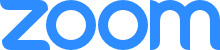#2021 SLA- Math 215 - Shared screen with speaker viewJulius Ngwa
33:04
Mean = 4.73Julius Ngwa
33:11
standard deviation = 1.13Julius Ngwa
35:00
Probability DistributionJulius Ngwa
38:59
Total Sample Size = 1000 HouseholdsJulius Ngwa
39:05
n = 1000Julius Ngwa
39:34
X = 0 (No Car) Number is 142Julius Ngwa
39:48
X = 1 (One Car) Number 378Julius Ngwa
40:03
X = 3 (Two Cars) Number is 423Julius Ngwa
40:20
X = 3 (Three Cars) Number is 57Julius Ngwa
40:41
142 + 378 + 423 + 57 = 1000Julius Ngwa
41:39
0 < P(x) < 1Julius Ngwa
41:46Julius Ngwa
41:57
Probability DistributionJulius Ngwa
42:04
Generated a Probability DistributionJulius Ngwa
42:22
Calculate the Mean as well as the Standard DeviationJulius Ngwa
57:29
.010Julius Ngwa
59:36
2nd EnterJulius Ngwa
01:00:29
P(X = 1) = .077Julius Ngwa
01:00:52
P(X = 2) = .230Julius Ngwa
01:01:14
P(X = 3) = .346Julius Ngwa
01:01:35
P(X = 4) = .259Julius Ngwa
01:01:55
P(X = 5) = .078Mary Holm
01:04:18
Mary HolmAshley Crockett
01:04:26
Ashley CrockettStacy
01:04:31
Stacy BolzeniusStacy
01:54:29
Thank youMary Holm
01:54:36